### NLP Solver Options

This section describes the options that are recognized by the NLP solver. These options can be specified after a forward slash (/) in the SOLVE statement, provided that the NLP solver is explicitly specified using a WITH clause.

#### Multistart Options

MSBNDRANGE=M

defines the range from which each variable can take values during the sampling process. This option affects only the sampling process that determines starting points for the local solver. It does not affect the bounds of the original nonlinear optimization problem. More specifically, if the ith variablehas lower and upper bounds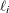and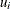respectively (that is,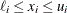), then an initial point is generated by a sampling process as follows:

For each sample point x, the ith coordinateis generated so that the following bounds hold where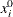is the default starting point or a specified starting point: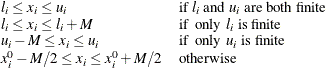This option is effective only when the MULTISTART option is specified. The default value is 200 in a shared-memory computing environment and 1,000 in a distributed computing environment.

MSDISTTOL=defines the tolerance by which two optimal points are considered distinct. Optimal points are considered distinct if the Euclidean distance between them is at least. This option is effective only when the MULTISTART option is specified. The default is=1.0E–6.

MSMAXTIME=T

defines the maximum allowable time T (in seconds) for the NLP solver to locate the best local optimum in multistart mode. The value of the TIMETYPE= option determines the type of units used. The time specified by the MSMAXTIME= option is checked only once after the completion of the local solver. Since the local solver might be called many times, the maximum time specified for multistart is recommended to be greater than the maximum time specified for the local solver (that is, MSMAXTIME
MAXTIME). This option is effective only when the MULTISTART option is specified. If you do not specify this option, the procedure does not stop based on the amount of time elapsed.

MSMAXSTARTS=N

defines the maximum number of starting points to be used for local optimization. That is, there will be no more than N local optimization calls in the multistart algorithm. You can specify N to be any nonnegative integer. When N = 0, the algorithm uses the default value of this option. In a shared memory computing environment, the default value is 100. In a distributed computing environment, the default value is a number proportional to the number of threads across all the grid nodes (usually more than 100). This option is effective only when the MULTISTART option is specified.

SEED=N

specifies a positive integer to be used as the seed for generating random number sequences. You can use this option to replicate results from different runs.

#### Optimization Options

ALGORITHM=keyword
TECHNIQUE=keyword
TECH=keyword
SOLVER=keyword

specifies the optimization technique to be used to solve the problem. The following keywords are valid:

INTERIORPOINT

uses a primal-dual interior point method. This technique is recommended for both small- and large-scale nonlinear optimization problems. This is the preferred solver if the problem includes a large number of inactive constraints.

ACTIVESET

uses a primal-dual active-set method. This technique is recommended for both small- and large-scale nonlinear optimization problems. This is the preferred solver if the problem includes only bound constraints or if the optimal active set can be quickly determined by the solver.

CONCURRENT (experimental)

runs the INTERIORPOINT and ACTIVESET techniques in parallel, with one thread using the INTERIORPOINT technique and the other thread using the ACTIVESET technique. The solution is returned by the first method that terminates.

The default is INTERIORPOINT.

MULTISTART
MS

enables multistart mode. In this mode, the local solver solves the problem from multiple starting points, possibly finding a better local minimum as a result. This option is disabled by default. For more information about multistart, see the section Multistart.

#### Output Options

LOGFREQ=N
PRINTFREQ=N

specifies how often the iterations are displayed in the SAS log. N should be an integer between zero and the largest four-byte, signed integer, which is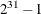. If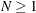, the solver prints only those iterations that are a multiple of N. If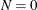, no iteration is displayed in the log. The default value is 1.

MSLOGLEVEL=number
MSPRINTLEVEL=number

defines the amount of information displayed in the SAS log by the MULTISTART option. Table 8.2 describes the valid values of this option.

Table 8.2: Values for MSLOGLEVEL= Option

number

Description

0

Turns off all solver-related messages to SAS log

1

Displays multistart summary information when the algorithm terminates

2

Displays multistart iteration log and summary information when the algorithm terminates

3

Displays the same information as MSLOGLEVEL=2 and might display additional information

This option is effective only when the MULTISTART option is specified. The default is 2.

SOLTYPE=01

specifies whether the NLP solver should return only a solution that is locally optimal. If SOLTYPE=0, the solver returns a locally optimal solution, provided it locates one. If SOLTYPE=1, the solver returns the best feasible solution found, provided its objective value is better than that of the locally optimal solution found. The default is 1.

#### Solver Options

FEASTOL=defines the feasible tolerance. The solver will exit if the constraint violation is less than FEASTOL and the scaled optimality conditions are less than OPTTOL. The default is=1E–6.

HESSTYPE=FULLPRODUCT

specifies the type of Hessian to be used by the solver. The valid keywords for this option are FULL and PRODUCT. If HESSTYPE=FULL, the solver uses a full Hessian. If HESSTYPE=PRODUCT, the solver uses only Hessian-vector products, not the full Hessian. When the solver uses only Hessian-vector products to find a search direction, it usually uses much less memory, especially when the problem is large and the Hessian is not sparse. On the other hand, when the full Hessian is used, the algorithm can create a better preconditioner to solve the problem in less CPU time. The default is FULL.

MAXITER=N

specifies that the solver take at most N major iterations to determine an optimum of the NLP problem. The value of N is an integer between zero and the largest four-byte, signed integer, which is. A major iteration in NLP consists of finding a descent direction and a step size along which the next approximation of the optimum resides. The default is 5,000 iterations.

MAXTIME=t

specifies an upper limit of t units of time for the optimization process, including problem generation time and solution time. The value of the TIMETYPE= option determines the type of units used. If you do not specify the MAXTIME= option, the solver does not stop based on the amount of time elapsed. The value of t can be any positive number; the default value is the positive number that has the largest absolute value that can be represented in your operating environment.

OBJLIMIT=M

specifies an upper limit on the magnitude of the objective value. For a minimization problem, the algorithm terminates when the objective value becomes less than –M; for a maximization problem, the algorithm stops when the objective value exceeds M. The algorithm stopping implies that either the problem is unbounded or the algorithm diverges. If optimization were allowed to continue, numerical difficulty might be encountered. The default is M=1E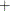20. The minimum acceptable value of M is 1E8. If the specified value of M is less than 1E8, the value is reset to the default value 1E20.

OPTTOL=RELOPTTOL=defines the measure by which you can decide whether the current iterate is an acceptable approximation of a local minimum. The value of this option is a positive real number. The NLP solver determines that the current iterate is a local minimum when the norm of the scaled vector of the optimality conditions is less thanand the true constraint violation is less than FEASTOL. The default is=1E–6.

TIMETYPE=numberstring

specifies the units of time used by the MAXTIME= option and reported by the PRESOLVE_TIME and SOLUTION_TIME terms in the _OROPTMODEL_ macro variable. Table 8.3 describes the valid values of the TIMETYPE= option.

Table 8.3: Values for TIMETYPE= Option

number

string

Description

0

CPU

Specifies units of CPU time

1

REAL

Specifies units of real time

The Optimization Statistics table, an output of PROC OPTMODEL if you specify the PRINTLEVEL=2 option in the PROC OPTMODEL statement, also includes the same time units for Presolver Time and Solver Time. The other times (such as Problem Generation Time) in the Optimization Statistics table are always CPU times.

The default value of the TIMETYPE= option depends on the values of the NTHREADS= and NODES= options in the PERFORMANCE statement of the OPTMODEL procedure. See the section PERFORMANCE Statement in Chapter 4: Shared Concepts and Topics. for more information about the NTHREADS= and NODES= options.

If you set the value of the NODES= option to 0 and the value of the NTHREADS= option to 1, the default value of the TIMETYPE= option is CPU. Otherwise the default value of the TIMETYPE= option is REAL.